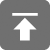# 工学1号馆

home

## Project Euler 15–Lattice paths

Wu Yudong    December 13, 2017     欧拉计划   555

Starting in the top left corner of a 2×2 grid, and only being able to move to the right and down, there are exactly 6 routes to the bottom right corner.How many such routes are there through a 20×20 grid?

#include<iostream>
#include<cmath>
using namespace std;

long long a;

int main()
{
int i;
for (i = 0; i < 21; i++) {
a[i] = 1;
a[i] = 1;
}
for (i = 1; i < 21; i++)
for (int j = 1; j < 21; j++)
a[i][j] = a[i][j - 1] + a[i - 1][j];
cout << a << endl;
return 0;
}